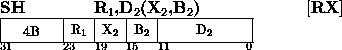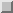Next: Subtract Logical Up: Insert Character Previous: Subtract

### Subtract halfwordThe second operand is subtracted from the first operand, and the difference is placed in the first-operand location. The second operand is two bytes in length and is considered to be a 16-bit signed integer.

The second operand is expanded to 32 bits before the subtraction by propagating the sign-bit value through the 16 high-order bit positions.

Subtraction is considered to be performed by adding the one's complement of the expanded second operand and a low-order one to the first operand. All 32 bits of both operands participate, as in ADD. If the carry out of the sign-bit position and the carry out of the high-order numeric bit position agree, the difference is satisfactory; ir they disagree, an overflow' occurs. The overflow causes a program interruption when the fixed-point overflow mask bit is one.

Resulting Condition Code:
0 ~ Difference is zero
1 ~ Difference is less than zero
2 ~ Difference is greater than zero
3 ~ Overflow

Program Exceptions:
Access (fetch, operand 2)
Fixed-Point Overflow

Programming Note
See Extended Mnemonic Instructions on pageNext: Subtract Logical Up: Insert Character Previous: Subtract

A.Daviel,TRIUMF